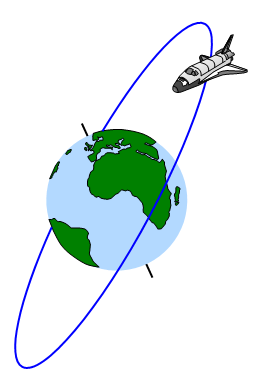# Kinetic energy in an orbitThe kinetic energy of an object in orbit can easily be found from the following equations:
Centripetal force on a satellite of mass m moving at velocity v in an orbit of radius r = mv2/r

But this is equal to the gravitational force (F) between the planet (mass M) and the satellite:

F =GMm/r2 and so mv2 = GMm/r

But kinetic energy = ˝mv2 and so:
kinetic energy of the satellite = ˝ GMm/r

Kinetic energy in orbit = ˝ mv2 = + ˝GMm/r

All satellites have to be given a tangential velocity (v) to maintain their orbit position and this process is called orbit injection.

Total energy of satellite in orbit = -GMm/2r

However the total energy INPUT required to put a satellite into an orbit of radius r around a planet of mass M and radius R is therefore the sum of the gravitational potential energy (GMm[1/R-1/r]) and the kinetic energy of the satellite ( ˝GMm/r).

Energy of launch = GMm[1/R – 1/2r]

Example problem
Calculate the total energy required to place the space shuttle in orbit.

Mass of space shuttle = 1.18x105 kg
Gravitational constant G = 6.67x10-11 Nm2kg-2
Mass of the Earth = 6x1024 kg    Radius of the Earth = 6.4x106 m
Velocity in this orbit: v = √GM/r = √[6.67x10-11x6x1024]/6.76x106 = 7690 ms-1
Total energy required = GMm[1/R –1/2r] = 3.9x1012 J

Note: this is very much simplified calculation. The mass used is just that of the shuttle orbiter. To raise the orbiter into its orbit requires rockets and fuel and these in turn need energy to lift them off the ground. The actual total energy required for the mission is therefore much larger than the result quoted above.

A VERSION IN WORD IS AVAILABLE ON THE SCHOOLPHYSICS CD